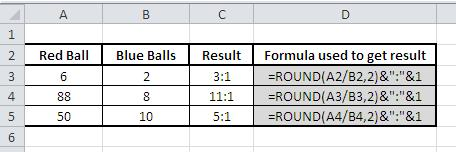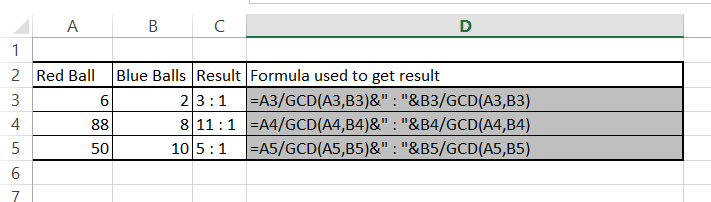### How To Calculate Ratio In Excel

Ratio: In simple mathematics, relationship or comparison between two more numbers is known as ratios. Ratios are normally written as “:” to show the connection between two numbers, for instance. If there are 2 red balls and 3 blue ball on the table you could write there ratio as: [2:3]

Unfortunately there is no systematic way to calculate ratio, but there is an easy way around for doing the same, you can try the below formulas to calculate ratio in Excel.

## How to calculate ratio using ROUND function?ROUND function in excel is used to trim down (reduce) a given value to a specific number of decimal places.

= ROUND (Number, Num_digits )

ROUND function will round up or down depending on whether the last digit is greater than or less than 5.

CONCATENATE function joins text strings into one text string. The joined items can be text, numbers, cell references, or a combination of those items. For example, if your worksheet contains a person’s first name in cell A1 and the person’s last name in cell B1, you can combine the two values in another cell by using the following formula:

= CONCATENATE (A1,” “, B1)

The second argument in this example (” “) is a space character. You must specify any spaces or punctuation that you want to appear in the results as an argument that is enclosed in quotation marks.

NOTE: You can also use the ampersand (&) calculation operator instead of the CONCATENATE function to join text items.

For example, =A1 & B1 returns the same value as =CONCATENATE (A1, B1).

## How to calculate ratio using GCD function?

There is also a method using GCD function. GCD stands for Greatest Common Divisor.

Finding the Greatest Common Divisor is the base of the formula. To calculate ratio you just need to divide two numbers by their GCDs.

The formula I used in the example:

=A3/GCD(A3,B3)&” : “&B3/GCD(A3,B3)Calculating ratio using GCD function is the most convenient method.

## How to calculate ratio using textfunctions?

I’m not sure if you will make me a serious. There is also a third method of finding ration in Excel. This is rather a bonus of this article. I doubt anyone will use that but it is working fine.

The formula I used: =SUBSTITUTE(TEXT(A3/B3,”#####/#####”),”/”,”:”)I don’t like calculating ratio using text functions. I am rather old fashioned and I’m using Excel rather for calculations and financial staff than manipulating strings by text functions.

## Template

```Further reading: